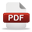## Learn

### Forward flux sampling

In this lesson we describe an real physical-experiment example.

The idea of the experiment:

Rare events are dificult to study, because of the long waiting times between their occurrences. Therefore, a lot of sampling techniques have been developed recently. However, the implementation of these techniques together with a simulation tool and calculating on high performance computers turned out to be very dificult. In this experiment we describe one of these samplinge techniques, namely Forward Flux Sampling (FFS) as a workow application in the context of the Science Experimental Grid Laboratory (SEGL). SEGL handles the workflow and dataow of the simulation, and matches the requirements for FFS by drawing a certain number of random datasets from a given dataspace. We demonstrate the usability of this work with an example simulation problem from physics. With this combination, FFS can easily be used together with any simulation software which can be described in the context of the SEGL framework, without the need of implementing the FFS method by the researcher himself, making rare event simulations easily available. Beyond that, trajectory fragments can be calculated in parallel and on high performance computing hardware by design.

Therefore, we focus on the forward flux sampling (FFS) approach which we apply to an example problem from physics, namely the barrier crossing of a particle. In FFS, the progress of a reaction from the initial state to the final state is characterized by an order parameter. The region between these two states is subdivided by several interfaces, which are positioned at certain values of the order parameter. Then, the simulation is driven step-wise towards the final state by first firing trajectories from the initial state and storing configurations, if they reach the next interface. From these configurations, new trajectories are red and so on, until the final state is reached. From the fraction of successful trajectories, the success probabilities can be determined, which, together with the flux of the trajectories which managed to leave the initial state in a certain simulation time, give the transition rate from the initial state to the final state. Beyond the transition rate, successful transition trajectories can be extracted from such a simulation, e.g. to investigate reaction pathways.

More details about the experiment you will find hereNow we may start the video lesson.